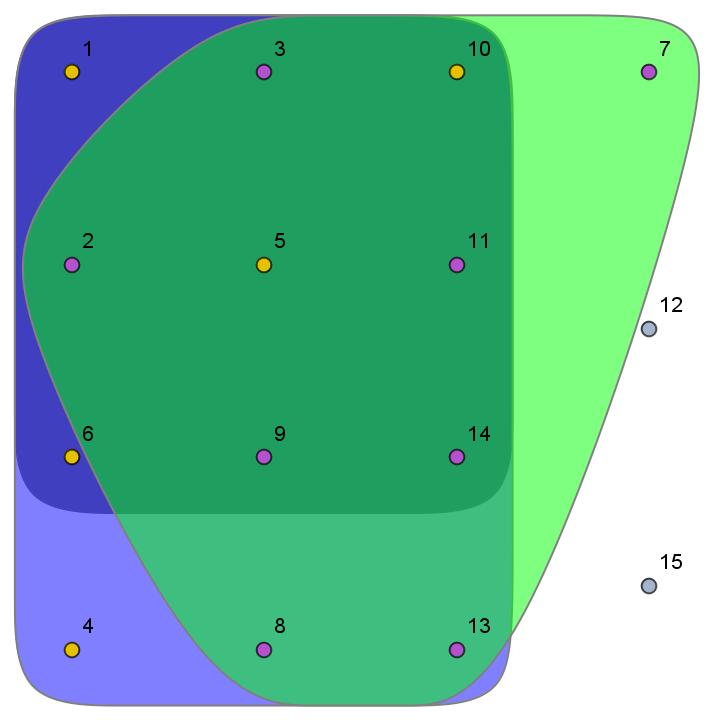# HypergraphPlot

Contributed by: Daniel McDonald

A plot of the hypergraph defined by a list of hyperedges and isolated vertices

 ResourceFunction["HypergraphPlot"][list] plots the hypergraph defined by list.

## Details and Options

The hypergraph represented by {{v11,v12,…},{v21,v22,…},…,u1,u2,…} has vertices v11,v12,…,v21,v22,…,…,u1,u2,… and hyperedges {v11,v12,…},{v21,v22,…},….

## Examples

### Basic Examples

Plot the hypergraph having vertices a,b,c,d,e,f,g and hyperedges {a,b,c,d},{b,c},{c,d,e}:

 In:=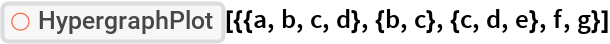Out=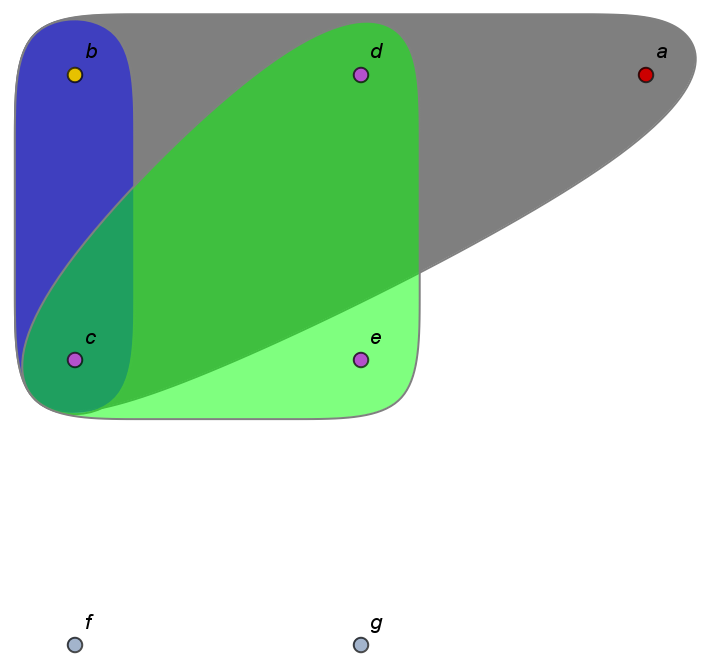### Applications

Define a random hypergraph having n vertices and m hyperedges:

 In:=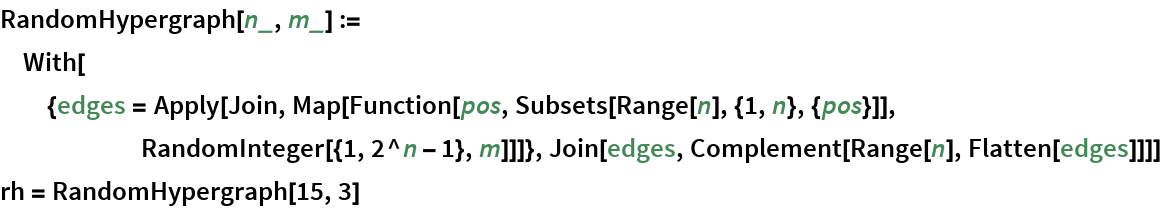Out=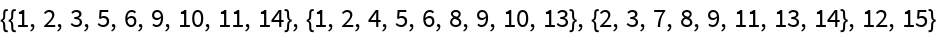Plot it:

 In:=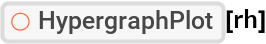Out=